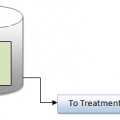## Coagulation and Flocculation Calculations

COAGULATION AND FLOCCULATION CALCULATIONS

Calculations are performed during operation of the coagulation and flocculation unit processes to determine chamber or basin volume, chemical feed calibration, chemical feeder settings, and detention time.
CHAMBER AND BASIN VOLUME CALCULATIONS
To determine the volume of a square or rectangular chamber or basin, we use:
Volume (cu ft) = length (ft) x width (ft) x depth (ft)
Volume (gal) = length (ft) x width (ft) x depth (ft) x 7.48 gal/cu ft

Example 16.1
Problem
A flash mix chamber is 4 ft square with water to a depth of 3 ft. What is the volume of water (in gallons) in the chamber?
Solution
Referring to Equation
Volume (gal) = 4 ft x 4 ft x 3 ft x 7.48 gal/cu ft
= 359 gal## Author:T.P.Sivanandan

#### Why Fine Bubbles are Better than Coarse bubble in Aeration Tank#### How to Calculate Lime Dosage Requirement in Sedimentation Process